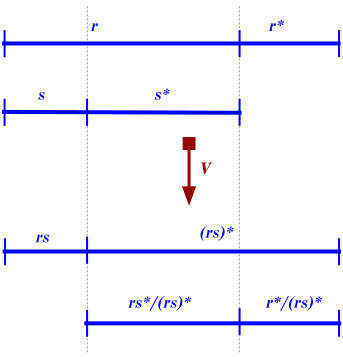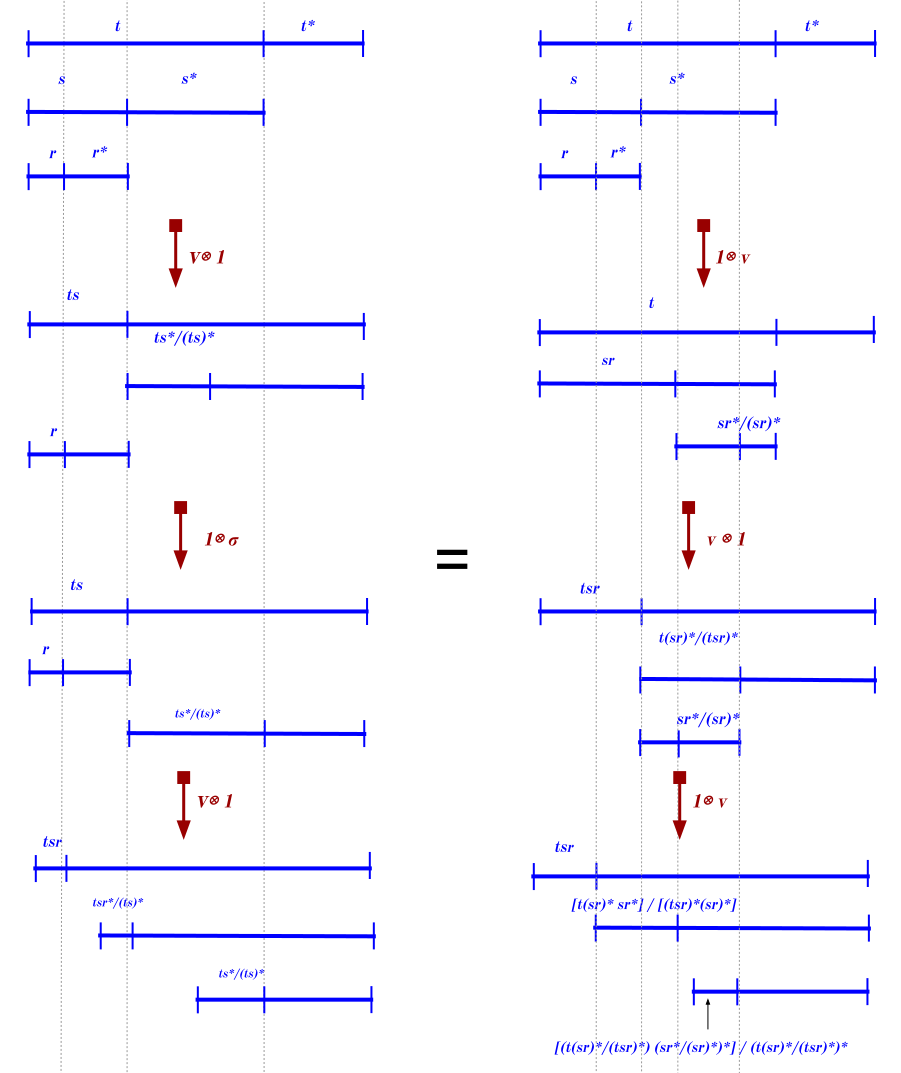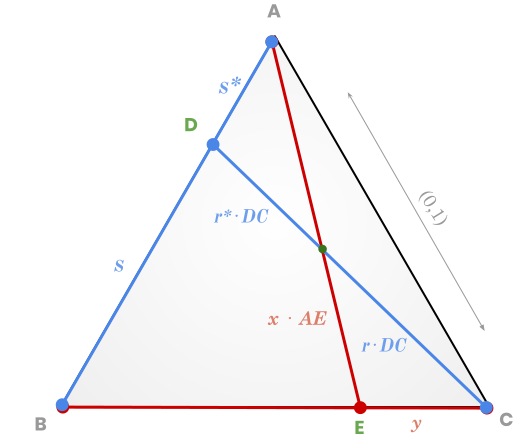# Tricocycloids, Effect Monoids & Effectuses#### Supervised by:   Richard Garner

Sydney,   April - August 2019

# Working Environment

## Centre Of Australian Category Theory (CoACT)Richard Garner
My Supervisor, Senior LecturerSteve Lack
Director, Associate ProfessorRoss Street
Founding Director, Emeritus ProfessorDominic Verity
Associate Director, Professor

## Centre Of Australian Category Theory (CoACT)

Tom Leinster calls them "ninja category theorists"

« One of my early Honours students at Macquarie University baffled his proposed Queensland graduate studies supervisor who asked whether the student knew the definition of a topological space. The aspiring researcher on dynamical systems answered positively:
"Yes, it is a relational $β$-module!"
I received quite a bit of flak from colleagues concerning that one; but the student Peter Kloeden went on to become a full professor of mathematics in Australia then Germany. »

— Ross Street (An Australian conspectus of higher categories)

# I. The distribution monad $\small {\D M}$

Shown to be a linear exponential monad Garner's 2018 "Hypernormalisation" article.

In what follows: $M ≝ [0, 1] ⊆ ℝ$ and $\;\ovee ≝ +$

Discrete distribution monad $\; \D M：\Set → \Set$:

• \begin{align*} \D M(X) &≝ \Big\lbrace φ：X → M \;\mathrel{\Big|}\;\supp(φ) \text{ finite and } \bigovee\limits_{x ∈ X} φ(x) = 1\Big\rbrace\\ &= \bigg\lbrace {\color{orange} \sum\limits_{i = 1}^n r_i \Ket{x_i}} \;\mathrel{\Big|}\; x_i ∈ X, \, r_i ∈ M, \, \bigovee\limits_{i} r_i = 1\bigg\rbrace \end{align*}\\
• $$η_X(x) ≝ 1 \Ket x \qquad\qquad μ_X\Big(\sum\limits_{i = 1}^n r_i \Ket{φ_i}\Big) ≝ \sum\limits_{x ∈ X} \Big(\bigovee\limits_{i=1}^n r_i ⋅ φ_i(x)\Big) \Ket x$$

Garner: Linear exponential monads are the perfect setting for hypernormalisation.

## What is so special about the unit interval?

which is related to... and leads to...
$[0,1]$ is an effect monoid quantum mechanics foundations $\D {[0, 1]}$ being a monad
$(0,1)$ is a tricocycloid quantum algebra $\D {[0, 1]}$ being $\underbrace{\text{linear exponential}}_{\llap{\text{related to linear logic} \, ⟶ \, \textbf{quantum }\text{logic}}}$
$\Kl(\D {[0, 1]})$ is an effectus quantum logic/computation $\EM(\D {[0, 1]}) ≅ \Conv {[0, 1]}$ in a state-and-effect triangle

⟹ How do these three notions relate?

## Quick categorical recap

### Kleisli category $\Kl(T)$ of a monad $T：𝒞 → 𝒞$

$\Kl(T)$:
• objects: $X ∈ 𝒞$
• morphisms: $X \xto f T(Y)$ for every $X \xto f Y ∈ 𝒞$

If $𝒞$ has coproducts $+$, so does $\Kl(T)$

Notation: $\; \KlN(T) ⊆ \Kl(T)$:
full subcategory whose objects are of the form $n ≝ 1 + ⋯ + 1 ∈ \Kl(T)$

### Eilenberg-Moore category/category of algebras $\; \EM(T)$

$\EM(T)$:
• objects: $(X, T(X) \xto {ξ} X ∈ 𝒞)$ such that $ξ ∘ μ_X = ξ ∘ T ξ$ and $\; ξ ∘ η_X = \id[X]$
• morphisms $(X, ξ) \xto f (Y, ν)$: $X \xto f Y ∈ 𝒞$ such that $f ∘ ξ = ν ∘ T(f)$

## II. What makes $\small {\D M}$ a monad?

$\D M$ being a monad:
we only use the fact that $M ≝ [0,1]$ is an effect monoid $M ∈ \EMon$, i.e. a monoid in the category $\EA$ of effect algebras.
Effect algebra $(E, \ovee, 0, (-)^⊥)$:
1. a Partial Commutative Monoid (PCM) where
• $\ovee ：E × E ⇀ E$ is a partial sum and $0 ∈ E$
• $\ovee\;$ is associative, commutative and satisfies the unit law (when defined)
2. equipped with an involution $(-)^⊥：E → E$ such that:
• $0$ is the unique element orthogonal to $1 ≝ 0^⊥$
• for all $x ∈ E$, $x^⊥$ is the unique element (orthocomplement) satisfying $x \,\ovee\, x^⊥ = 1$

$x_1, …, x_n ∈ M$ orthogonal: if their partial sum is defined. When $\; x, y ∈ M \text{ orthogonal}$: $x \,⊥\, y$.

Morphisms of effect algebras:
maps preserving the partial sum and $1$.

Effect Monoid $M ∈ \EMon ⊆ \EA$:
Effect algebra with an associative multiplication distributing over the partial sum and having $1$ as neutral element.

Effect Module $E ∈ \EMod M ≝ \EM(M ⊗ (-))$ over an effect monoid $M ∈ \EMon$:
Effect algebra $E$ with a scalar multiplication $⋅：M ⊗ E → E$ preserving $0$ and the partial sum coordinatewise and such that $1⋅e = e$ and $r⋅(s⋅e) = (r⋅s)⋅e$.

#### Effect algebras: arising in quantum logic

• Generalise both
• quantum effects: self-adjoint bounded linear operators on a Hilbert space (quantum observables) between $0$ and $\id$
• and orthomodular lattices
• abstraction of the lattice of closed subspaces of a Hilbert space (Von Neumann's experimental propositions about quantum observables)

« Dr. Von Neumann, I would like to know what is a Hilbert space? » — David Hilbert (at the end of a lecture given by Von Neumann in Göttingen in 1929)

Also generalise

• Boolean algebras (distributive complemented lattices)
• orthomodular lattices (quantum case): not distributive
• and $σ$-algebras
• Probability measures: morphisms in $\EA$

⟹ Can be thought of as generalising both propositions and probabilitiesCan we generalise from $\D {[0,1]}$ to a general $\D M$, where $M$ is an arbitrary effect monoid?

⟶ If so, do we still have a linear exponential monad?

## III. What makes $\small {\D M}$ linear exponential?

• Lifts a certain tensor product $\star_{(0,1)}$ making $(\Set, \star_{(0,1)})$ symmetric monoidal to a coproduct in $\EM(\D M)$

• in Linear Logic (LL): exponential modality $?$ turning additives into multiplicatives:
$$?(A ⊕ B) \; ≡ \; ?A ⅋ ?B$$
• One of the 3 ingredients to have a

Model of classical linear logic (MAELL):
1. M(ultiplicative fragment): Symmetric monoidal closed category (SMCC) which is $\ast$-autonomous
2. A(dditive fragment): Finite co/products
3. E(xponential fragment): Linear exponential co/monad

⟶ Linear logic: argued to be the "proper incarnation" of quantum logic (see Pratt's 1992 article)

« One of the wild hopes that this suggests is the possibility of a direct connection with quantum mechanics… but let’s not dream too much! » — Girard (Linear logic, 1987)

### III.1. Because ${\small (\Set, \star_{(0,1)})}$ is monoidal

What makes the tensor $A \star_{(0,1)} B ≝ A + (0,1) × A × B + B$ endow $\Set$ with a symmetric monoidal structure ⟶ the fact that $(0,1)$ is a tricocycloid in $\Set$

A tricocycloid in a symmetric monoidal category $(𝒞, ⊗, α, λ, ρ, σ)$:

is an object $H ∈ 𝒞$ with an isomorphism $v：H ⊗ H → H ⊗ H$
satisfying the 3-cocycle condition:

$$(v ⊗ 1)(1 ⊗ σ)(v ⊗ 1) = (1 ⊗ v) (v ⊗ 1)(1 ⊗ v)$$

A symmetry $γ$ for a tricocycloid $H$:
is an involution $γ：H → H$ such that
$$(1 ⊗ γ) v (1 ⊗ γ) = v (γ ⊗ 1) v$$

A tricocycloid with a symmetry: symmetric tricocycloid.

### Tricocycloids

• Quantum algebraic objects introduced by Street in 1998

• Generalisation of Hopf algebras
• generalising group algebras: bialgebra with an antipode
• ⟶ quantum groups
• 3-cocycle condition: $(v ⊗ 1)(1 ⊗ σ)(v ⊗ 1) = (1 ⊗ v) (v ⊗ 1)(1 ⊗ v)$

• non-abelian cohomology: geometrical nerve of the double suspension of the braided monoidal category seen as tricategory with one object and one morphismWhy such a cocycle condition? How is it related to the problem at hand?

Cocycles in non-abelian cohomology ⟷ coherence conditions in higher-dimensional categories (associativity for 3-cocycle).

Here: associativity/coherence condition: an axiom of abstract convex sets (objects of the category of algebras)

• Yang-Baxter equations: recurrent in quantum mechanics

Key lemma (Street and Garner): If $H ∈ 𝒞$ is a symmetric tricocycloid and $𝒞$ has finite coproducts $+$ over which $⊗$ distributes: we can co-universally make $(𝒞, ⋆_{H})$ a monoidal category (with $0$ as unit) by putting

$$A ⋆_{H} B ≝ A + H ⊗ A ⊗ B + B$$

### The unit interval is a tricocycloid in ${\small (\Set, ×)}$$v：(0,1)^2 → (0,1)^2$

$(0,1) ∈ \Set$ is a symmetric tricocycloid:
• $v ≝ \begin{cases} (0,1)^2 &→ (0,1)^2\\ (r,s) &↦ \Big(rs, \frac {rs^\ast}{(rs)^\ast}\Big) \end{cases}$

• $γ ≝ (-)^\ast = \begin{cases} (0,1) &→ (0,1)\\ r &↦ r^\ast ≝ 1-r \end{cases}$3-cocycle condition for $(0,1)$

### Geometric interpretation$v$ sends $(r, s)$ to $(x, y)$

### III.1. Because ${\small \EM(\D {[0,1]}) \,≅\, \Conv {[0,1]}}$Very ad hoc to $\D {[0,1]}$. What about other probability monads? ⟶ Way harder to generalise

but... $\Kl(\D {[0,1]})$ (and the Kleisli category other probability monads as well) happens to be an effectus! (Jacobs 2018)

Effectus theory:
young branch of categorical logic, developed by Jacobs, Cho and the Westerbaan brothers. Effectuses = categorical models capturing the fundamentals of discrete/continuous/quantum logic and probability.

$$\frac{\text{Effectus theory}}{\text{Quantum logic}} \; ≃ \; \frac{\text{Topos theory}}{\text{Intuitionistic logic}}$$

Effectus $𝔹$:
a category with a terminal object $1$ and finite coproducts $+$ which satisfies mild pullback assumptions and a joint monicity requirement so that, for all $X ∈ 𝔹$
• the set $\M ≝ \Hom[𝔹]{1, 2}$ of scalars forms an effect monoid
• the set $\Stat(X) ≝ \Hom[𝔹]{1, X}$ of states of $X$ forms an abstract convex set over $\M$
• the set $\Pred(X) ≝ \Hom[𝔹]{X, 2}$ of predicates over $X$ forms an effect module over $\M$

Examples of effectuses:

• $\Set$ (modelling classical computation and logic)
• the opposite category $\opposite \Cpu$ of $C^\ast$-algebras and positive unital maps (modelling quantum computation/logic)
• the opposite categories $\opposite \DL$ of distributive lattices, $\opposite \BA$ of boolean algebras, and $\opposite \Rng$ of rings
• Octavio Zapata 2019: $\Kl(\D M)$ is an effectus for $M$ is an effect monoid.

An effectus $𝔹$ induces a state-and-effect triangle:$\Kl(\D \M)$ is an effectus (Zapata 2019): how is it related to $𝔹$?

# Further results

• If $𝔹$ has finite coproducts of $1$ as objects, with $q = [q^1, …, q^m]：m → n$:

$$F ≝ \begin{cases} 𝔹 & \overset{≅}{⟶} \; \KlN(\D \M) \\ n &⟼ \; n\\ m \xto q n &⟼ \begin{cases} m &⟶ \; \D \M (n) \\ k &⟼ \; \displaystyle \smash{\sum\limits_{1 ≤ i ≤ n}} ⊳_i q^k \Ket i \end{cases} \end{cases}$$

from which we get

and $\Stat \text{ is fully-faithful } ⟺ \Nerve i \text{ is fully-faithful } ⟺ i \text{ is dense}$

• When $M$ has normalisation:

• objects in $\Conv M$ given by binary convex sums (generalises Garner's situation).
• $\D \M$-algebras are idempotent commutative $⋆_H$-monoids ⟹ $\D \M$ is linear exponential
• Jacobs: $\Stat$ preserves $+$ when $\M = [0,1]$ ⟶ what about a $\M$ with normalisation?
• Models of LL: does $\D M$ live in a model of linear logic?

• $(\Set, ⋆_{\mathring M})$ is a SMC, but $0$ is a unit for $⋆_{\mathring M}$ ⟹ can't be closed.

Do we have a model of $\text{MELL}^{-}$ (without units)? (for Girard's proof nets)

Houston 2013: defines a good notion of model of $\text{MLL}^{-}$. Criterion: every arrow $A → B$ stems from a unique linear element of $A ⊸ B$ (ie a $γ_X：X → (A ⊸ B) ⊗ X$ such that $α_{A ⊸ B, X, Y} (γ_X ⊗ Y) = γ_{X ⊗ Y}：X ⊗ Y → (A ⊸ B) ⊗ (X ⊗ Y)$).

BUT condition not met in our case.

• To fix $0$ being the unit for the tensor: how about $⋆_M$, where $M$ is seen as a lax tricocycloid? ⟶ Fails: Street's construction only yields a lax semi-monoidal category.

• If $F：\catA → \catB$ is lax monoidal ⟹ the left Kan extension $\Lan F：\Psh\catA → \Psh\catB$ can be shown to be lax monoidal. So if $T：\catA → \catB$ is an opmonoidal monad, $\opposite T： \opposite\catA → \opposite\catB$ is lax monoidal, and $\Lan F：[\catA, \Set] → [\catB, \Set]$ is lax monoidal, thus $\opposite{\Lan F}：\opposite{[\catA, \Set]} → \opposite{[\catB, \Set]}$ is oplax monoidal. If $T$ is linear exponential, does $\opposite{\Lan F}$ remain linear exponential?

# Conclusion